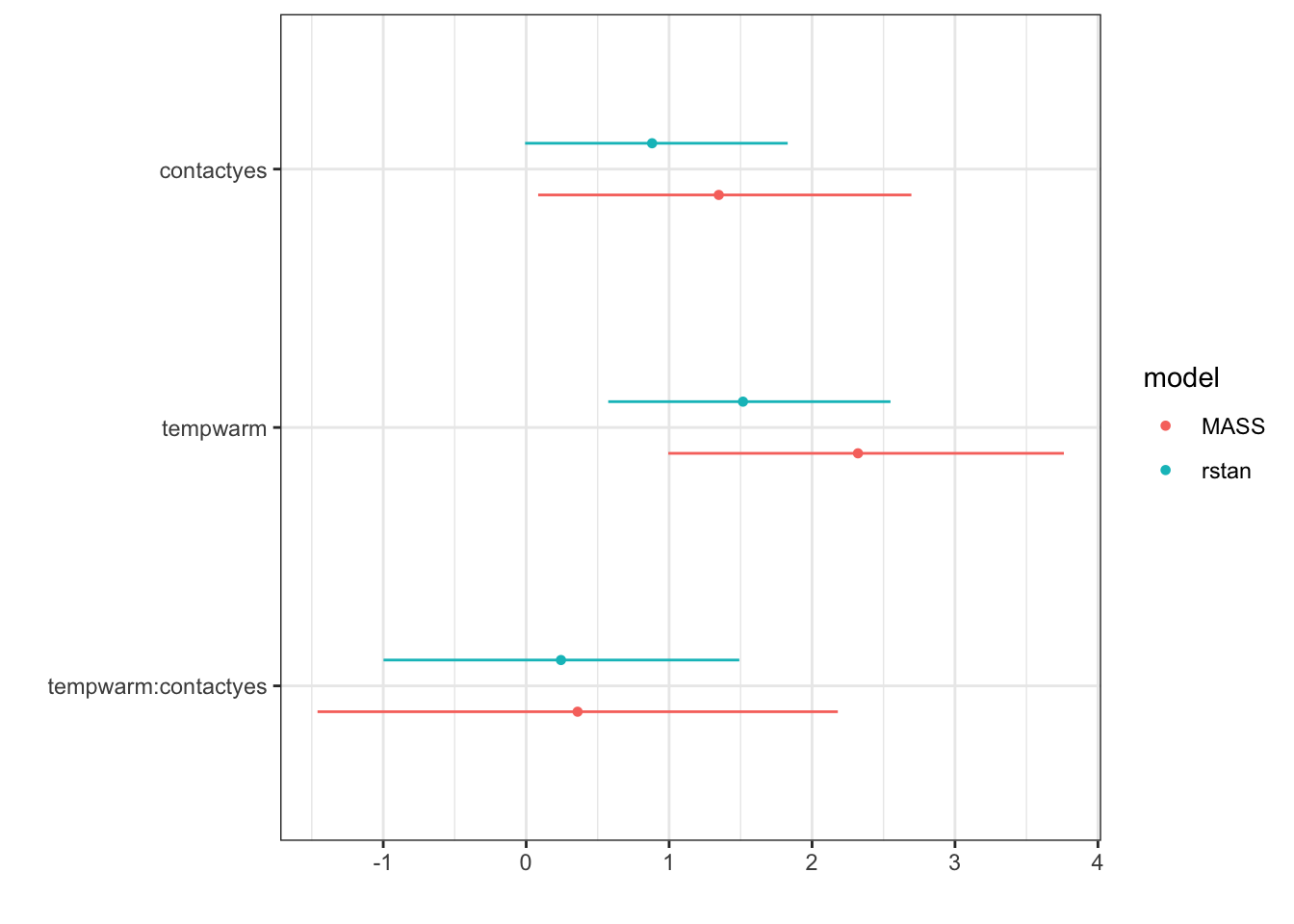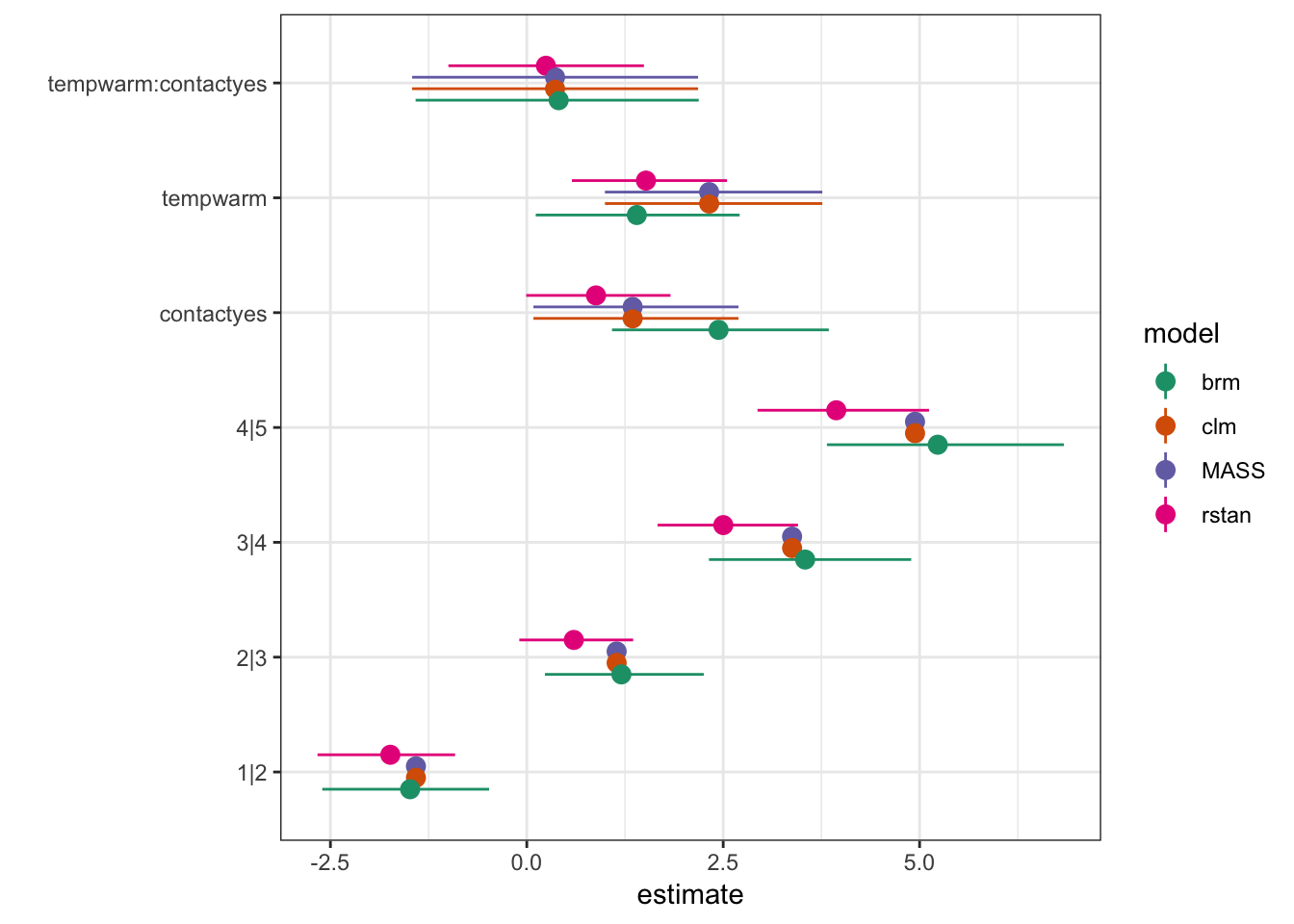# Introduction

Constructing confidence intervals for the predicted probabilities of ordinal models turns out to be surprisingly (to me) hard. The basic problem is that the standard cumulative link models predict cumulative probabilities, not probabilities. It’s easy to construct CIs for the cumulative probabilities by the standard methods: i.e. assuming MVN sampling distributions of parameters on the link scale, construct Normal CIs for the cumprob predictions and apply the inverse link function to get back to the [cumulative] probability scale). Unfortunately the probabilities themselves are differences of cumulative probabilities on the probability scale (not the link scale), so the standard tricks don’t work.

People ask this question a lot (e.g. here, here)

The only simple choices I can think of for standard frequentist approaches are

• some sort of delta-method approximation (these are dicey because they usually end up making a Normality assumption on the prediction scale, which can be bad: it’s conceivable that one exists that’s better than the one the `emmeans` package uses)
• a pseudo “prediction interval” (sensu Lande et al.) based on sampling random MVN values of the parameter vector
• Jonathan Dushoff points out that if you can be satisfied with effects plots that show the change in probability from a specified baseline and incorporate the uncertainty of only one predictor, this can be done in the classical framework.

The other choice is to use a Bayesian method, which is illustrated below.

# Model fitting

``````library(ordinal)
library(brms)
library(MASS)  ## polr: *before* dplyr
library(dplyr)
library(tidyr)
library(tidybayes)
library(rstanarm)
library(broom)
library(broom.mixed)
library(dotwhisker)
library(ggplot2); theme_set(theme_bw())``````

Fit the same model in `ordinal` and `brms` using the basic `wine` example from the `ordinal` package:

``````m_clm <- clm(rating ~ temp * contact, data = wine)
m_brm <- brm(rating ~ temp * contact,
family =cumulative(threshold="flexible"),
data = wine,
refresh=0   ## quiet
)``````

Add `MASS::polr` and `rstanarm::stan_polr` for comparison:

``````c_MASS <- polr(rating ~ temp * contact, data = wine)
c_rstan <- stan_polr(rating ~ temp * contact, data = wine,
prior=R2(0.2,"mean"),
refresh=0)``````

Could also try this with `MCMCglmm`.

Trying to do a dot-whisker plot to compare parameters (but some technical problems …)

``````fitlist <- list(clm=m_clm,brm=m_brm,MASS=c_MASS,
rstan=c_rstan)
## FIXME: tidy() has problems with m_brm (bad 'term' var)
## FIXME: dwplot() has problems with m_clm
dwplot(fitlist[3:4])``````Hack our own dot-whisker plot (don’t need `ggstance::geom_pointrangeh` because we’re not trying to facet, you we can `coord_flip()` instead)

``````tdf <- purrr::map_dfr(fitlist,tidy,.id="model",conf.int=TRUE)
## hack to fix brm term names
tdf[tdf\$model=="brm","term"] <- tdf[tdf\$model=="clm","term"]
pd <- position_dodge(width=0.4)
(ggplot(tdf,aes(x=term,y=estimate,colour=model))
## redundant (some models missing intercept CIs)
+ geom_point(position=pd,size=3)
+ geom_pointrange(aes(ymin=conf.low,ymax=conf.high),
position=position_dodge(width=0.4))
+ coord_flip()
+ scale_colour_brewer(palette="Dark2")
+ labs(x="")
)``````Slightly different predictions (median vs MLE; priors in rstan and brms).

## predictions with CIs

Prediction data frame (for marginal values):

``````pframe <- with(wine,expand.grid(temp=levels(temp),
contact=levels(contact)))``````

Reorg/gather predictions from both approaches:

``````pred_clm <- (data.frame(pframe,
setNames(as.data.frame(predict(m_clm,newdata=pframe)),1:5),
check.names=FALSE)
%>% tidyr::gather(rating,est,-c(temp,contact), factor_key=TRUE)
)

pred_brm <- (tidybayes::fitted_draws(m_brm,pframe,category="rating")
%>% select(temp,contact,rating,.value)
%>% group_by(temp,contact,rating)
%>% summarise(lo=quantile(.value,0.025),
est=median(.value),
hi=quantile(.value,0.975))
%>% ungroup
)``````
``## Adding missing grouping variables: `.row```
``pred_all <- dplyr::bind_rows(list(clm=pred_clm,brm=pred_brm), .id="model")``

Draw the picture:

``````gg0 <- (ggplot(pred_all,aes(temp,est,colour=rating))
+ geom_point(aes(shape=model))
+ geom_line(aes(group=interaction(model,rating),linetype=model))
## NAs in clm predictions mess this up, so restrict to pred_brm
+ geom_ribbon(data=pred_brm,aes(ymin=lo,ymax=hi,
fill=rating,group=rating),
colour=NA,alpha=0.2)
)
gg0 + facet_wrap(~contact, labeller=label_both)``````